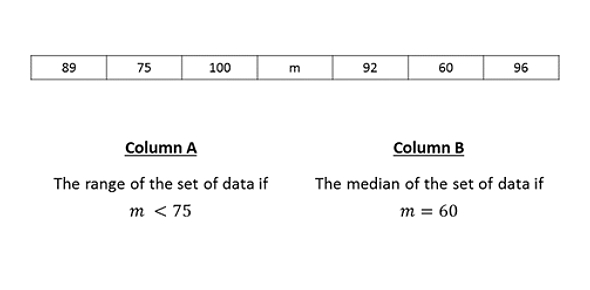# Advanced SCAT Mini (G6 And Above) - Math

10 Questions | Total Attempts: 3885SettingsEach of the following questions has two parts. One part is in Column A. The other part is in Column B. You must find out if one part is greater than the other, if the parts are equal, or if not enough information is given for you to decide. Then, choose one of the four answers below: (A) if the part in Column A is greater (B) if the part in Column B is greater (C) if the two parts are equal (D) if not enough information is given to decide Note: Letters such as x, n, and k stand for real numbers. If the same letter appears in both columns of a question, it stands for the same

• 1.

• A.

The part in Column A is greater

• B.

The part in Column B is greater

• C.

The two parts are equal

• D.

Not enough information is given to decide

• 2.

• A.

The part in Column A is greater

• B.

The part in Column B is greater

• C.

The two parts are equal

• D.

Not enough information is given to decide

• 3.

• A.

The part in Column A is greater

• B.

The part in Column B is greater

• C.

The two parts are equal

• D.

Not enough information is given to decide

• 4.
• A.

The part in Column A is greater

• B.

The part in Column B is greater

• C.

The two parts are equal

• D.

Not enough information is given to decide

• 5.
• A.

The part in Column A is greater

• B.

The part in Column B is greater

• C.

The two parts are equal

• D.

Not enough information is given to decide

• 6.
• A.

The part in Column A is greater

• B.

The part in Column B is greater

• C.

The two parts are equal

• D.

Not enough information is given to decide

• 7.
• A.

The part in Column A is greater

• B.

The part in Column B is greater

• C.

The two parts are equal

• D.

Not enough information is given to decide

• 8.
• A.

The part in Column A is greater

• B.

The part in Column B is greater

• C.

The two parts are equal

• D.

Not enough information is given to decide

• 9.
• A.

The part in Column A is greater

• B.

The part in Column B is greater

• C.

The two parts are equal

• D.

Not enough information is given to decide

• 10.
• A.

The part in Column A is greater

• B.

The part in Column B is greater

• C.

The two parts are equal

• D.

Not enough information is given to decide

Related Topics